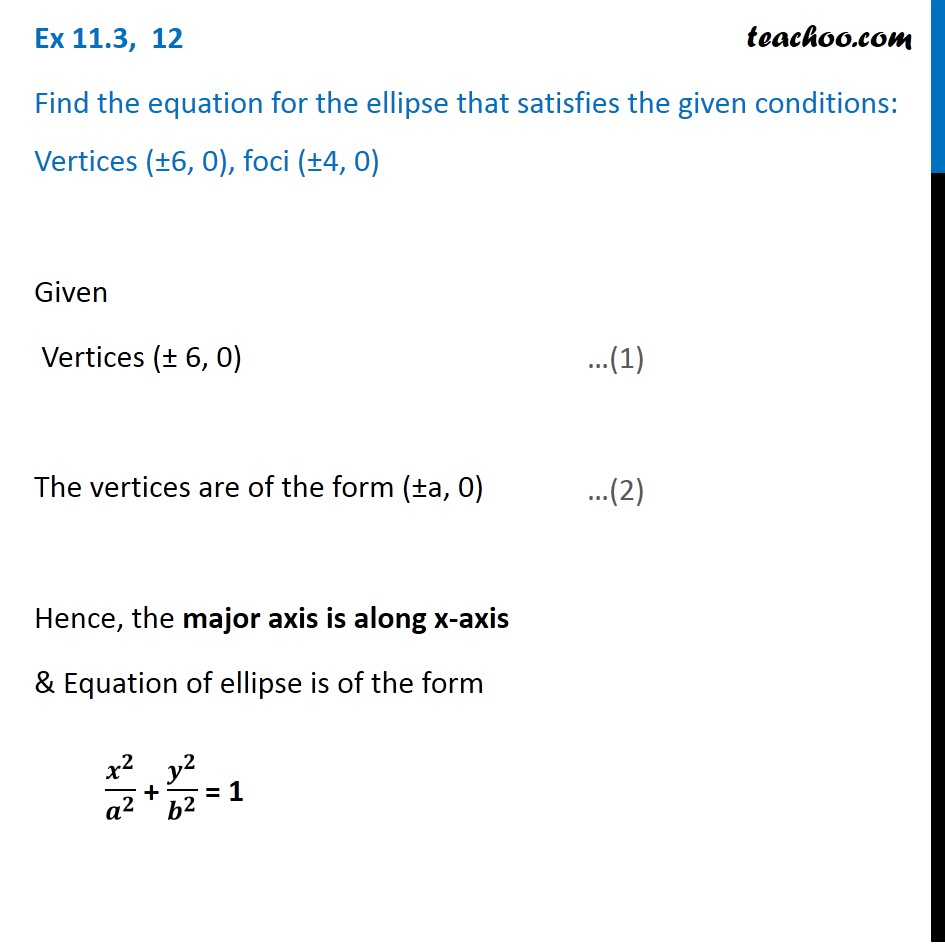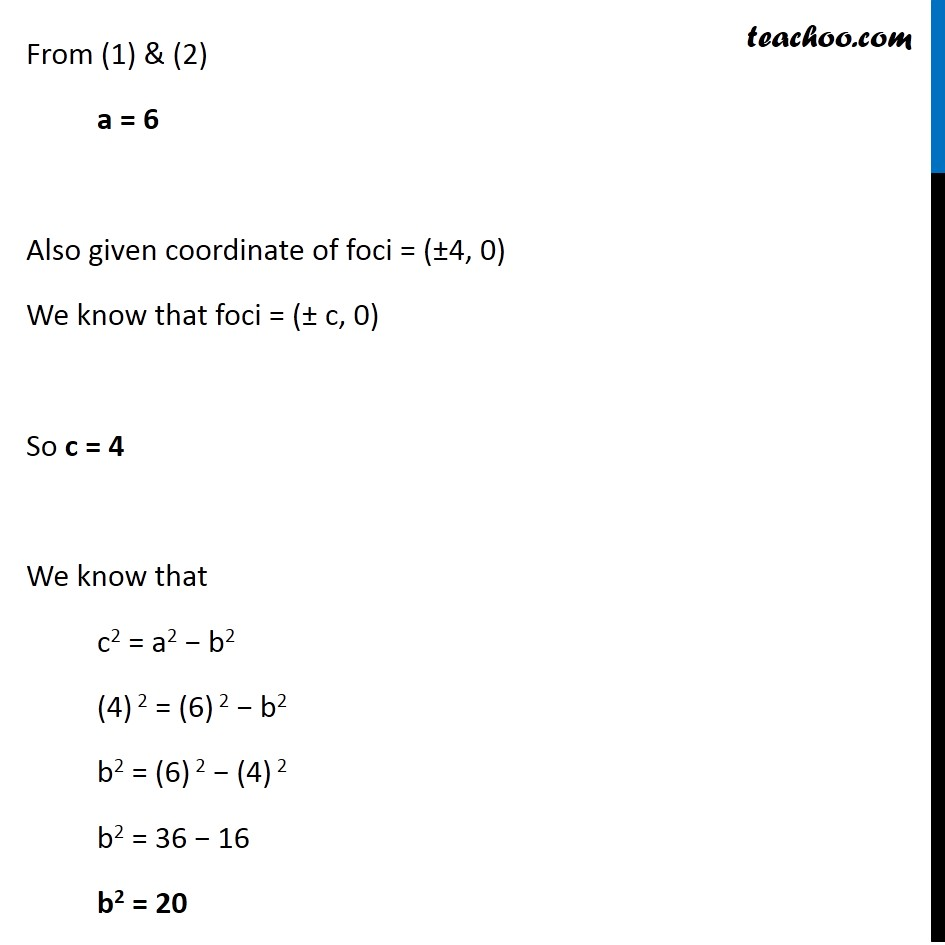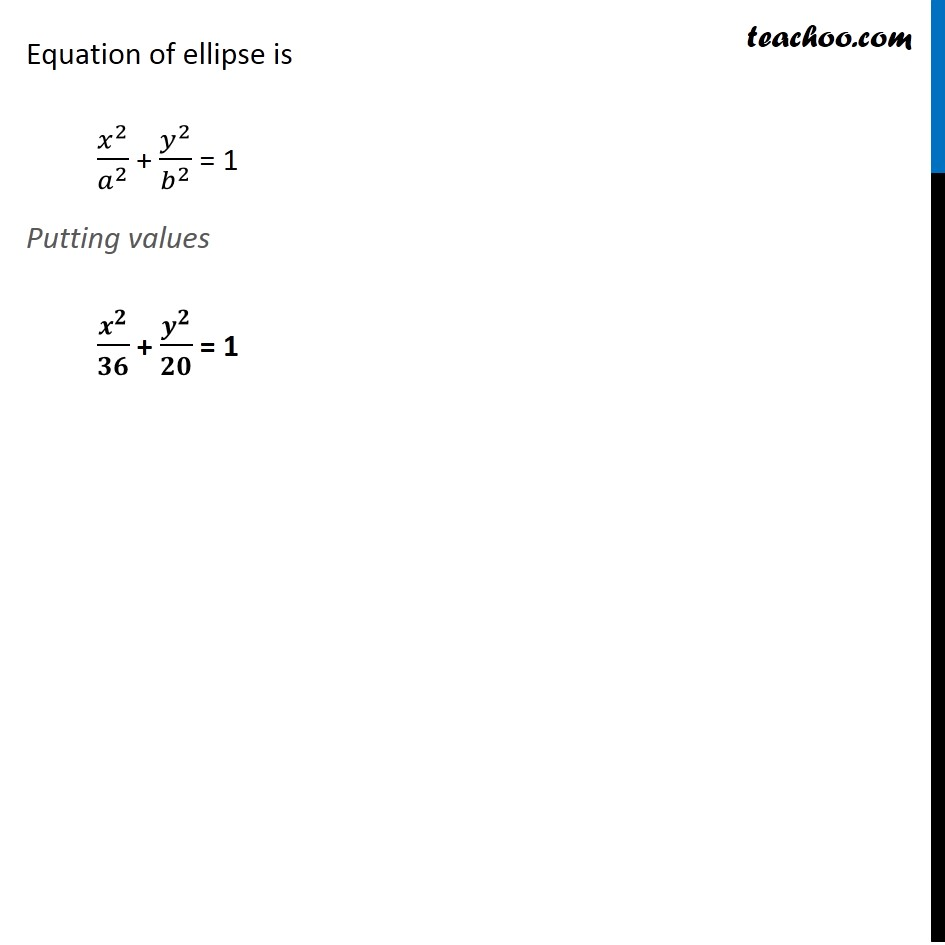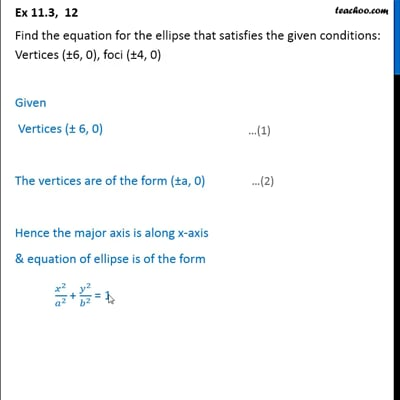Ex 11.3

Chapter 11 Class 11 Conic Sections (Term 2)
Serial order wiseThis video is only available for Teachoo black users

### Transcript

Ex 11.3, 12 Find the equation for the ellipse that satisfies the given conditions: Vertices (±6, 0), foci (±4, 0) Given Vertices (± 6, 0) The vertices are of the form (±a, 0) Hence, the major axis is along x-axis & Equation of ellipse is of the form 𝒙^𝟐/𝒂^𝟐 + 𝒚^𝟐/𝒃^𝟐 = 1 From (1) & (2) a = 6 Also given coordinate of foci = (±4, 0) We know that foci = (± c, 0) So c = 4 We know that c2 = a2 − b2 (4) 2 = (6) 2 − b2 b2 = (6) 2 − (4) 2 b2 = 36 − 16 b2 = 20 Equation of ellipse is 𝑥^2/𝑎^2 + 𝑦^2/𝑏^2 = 1 Putting values 𝒙^𝟐/𝟑𝟔 + 𝒚^𝟐/𝟐𝟎 = 1